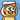# An Elegant Way to Solve Adding Commas Between Every 3 Digits Problem in JavaScript

April 09, 2019

While browsing Zhihu earlier today, I saw some front-end interview questions from those big tech companies here web 前端大厂 10 道经典面试题汇总（含答案详解）

The first question caught my attention.

Write a JavaScript function to patch a comma symbol between every 3 digits of a positive number. e.g. Input 100000, Output 100,000

This is a quite simple algorithm question. However, when I saw the provided solution, I felt it was not “JavaScript” enough.

Here is the provided solution (Considering readability, I have formatted the code to my own coding style… LOL):

```var arr = []; function main(num) { if (num === null) return; var n = parseInt(num).toString(); s(n); } function s(num) { if (num.length > 3) { arr[arr.length] = num.slice(-3); s(num.slice(0, -3)); } else { arr[arr.length] = num; } } main(123456789); console.log(arr.reverse().join(","));```

The solution used 2 functions to solve the problem, which I think is unnecessary.

The target is simple, just to take a number in and output a processed string. Hence, I think one function would be more than enough.

Besides, the function used `if` to control the logic `num.length > 3`, and if so, it will recursively call itself to operate on the number string, which I think is very tedious and unnecessary. From the view point of software engineering quality attribute, I think the code readability and maintainability are not good.

Thanks to EcmaScript 6 standard, JavaScript now has quite a number of Array utility functions. We can actually make use of these features to write more comprehensive codes to elegently solve our problem.

Here is my improved solution to the problem:

```function addComma(num) { if (num === null) return; return ( num .toString() // transform the number to string .split("") // transform the string to array with every digit becoming an element in the array .reverse() // reverse the array so that we can start process the number from the least digit .map((digit, index) => index != 0 && index % 3 === 0 ? `\${digit},` : digit ) // map every digit from the array. // If the index is a multiple of 3 and it's not the least digit, // that is the place we insert the comma behind. .reverse() // reverse back the array so that the digits are sorted in correctly display order .join("") ); // transform the array back to the string } console.log(addComma(123456789)); // Output: 123,456,789```

If you have worked with RxJs, you may probably be very familiarized with this style. In this case, you are seeing the `num` as a data stream and then process it with chaining functions. It may be difficult to understand in the beginning, but it’s actually comprehensive as you have a clear mind in what steps you have taken to manipulate the object.

Definitely it’s much easier to understand comparing to `if-else` logic with recursive functions.

That is the beauty of JavaScript. With native array utility functions, you can make your logic simple and clear.Written by Yi Zhiyue
A Software Engineer · 山不在高，有仙则灵